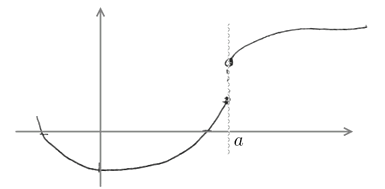# ML Wiki

## Continuous Functions

A Function $f(x)$ is continuous if the Limit of this function always exists otherwise the function is discontinuous

• $f(x)$ is continuous at $x = a$ if $\lim\limits_{x \to a} f(x) = f(a)$
• $f(x)$ is continuous everywhere if its continuous for all $a$ in the domain of $f(x)$

Many functions are continuous, for example:

Careful!

• some functions might look discontinuous, but they may be continuous
• this is the case when the discontinuously-looking points are not in the domain
• but if the function is defined in this point, then it's discontinuous

Discontinuous: